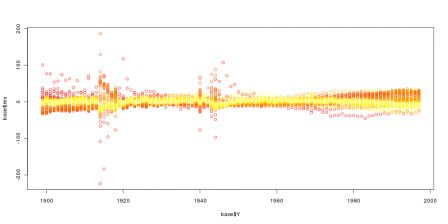Want to share your content on R-bloggers? click here if you have a blog, or here if you don't.

Last course will be uploaded soon (the links will be here and there). The R code considered is given below. First, we had to work a little bit on the datasets,

```tabB=read.table("http://perso.univ-rennes1.fr/arthur.charpentier/tabB.csv",
ANNEE=tabB[,1]
BASEB=tabB[,seq(2,246,by=2)]
BASEB=as.matrix(BASEB[,1:100])
AGE=0:ncol(BASEB)
an=tabC[,1]
an=an[-c(16,23,43,48,51,53,106)]
BASEC=tabC[,2:101]
BASEC=as.matrix(BASEC[-c(16,23,43,48,51,53,106),])
BASEB=BASEB[,1:90]
BASEC=BASEC[,1:90]
AGE=AGE[1:90]
MU=as.matrix(log(BASEB/BASEC))
col="green")
library(rgl)
persp3d(ANNEE,AGE,MU,col="light blue")```
(this last line is here to play a little bit with the 3d mortality surface). We first used the Lee-Carter function proposed by JPMorgan in LifeMetrics,
```source("http://perso.univ-rennes1.fr/arthur.charpentier/fitModels.r")
x=AGE
y=ANNEE
d=BASEB
e=BASEC
w=matrix(1,nrow(d),ncol(e))
LC1=fit701(x,y,e,d,w)
plot(AGE,LC1\$beta1)
plot(ANNEE,LC1\$kappa2)
plot(AGE,LC1\$beta2)```
Then we considered nonlinear Poisson regression,
```D=as.vector(BASEB)
E=as.vector(BASEC)
A=rep(AGE,each=length(ANNEE))
Y=rep(ANNEE,length(AGE))
base=data.frame(D,E,A,Y,a=as.factor(A),
y=as.factor(Y))

LC2=gnm(D~a+Mult(a,y),offset=log(E),
family=poisson,data=base)
plot(AGE[-1],LC2\$coefficients+LC2\$coefficients[2:90])
lines(AGE,LC1\$beta1,col="blue")
plot(ANNEE,LC2\$coefficients[181:279])
plot(ANNEE,LC1\$kappa2,col="red")
plot(AGE,LC1\$beta2)```
As mentioned during the course, this technique is great… but it is sentive to initial values in the optimization procedure. For instance, consider the following loops,
```plot(AGE[-1],LC2\$coefficients+LC2\$coefficients[2:90],
type="l",col="blue",xlab="",ylab="")
for(s in 1:200){
LC2=gnm(D~a+Mult(a,y),offset=log(E),
family=poisson,data=base)
lines(AGE[-1],LC2\$coefficients+LC2\$coefficients[2:90],col="blue")
}```
Here are representation of the first component in the Lee-Carter model,Hence, the estimation depends on the choice of initial values… even if the shape remains unchanged…
Then, we finnished with Rob Hyndman’s package,
```library(demography)
base=demogdata(data=t(exp(MU)),pop=t(BASEC),
ages=AGE,years=ANNEE,type="mortality",
label="France",name="Total",lambda=0)
LC3=lca(base)
LC3F=forecast(LC3,100)
plot(LC3\$year,LC3\$kt,xlim=c(1900,2100),ylim=c(-300,150))
lines(LC3F\$year,LC3\$kt+LC3F\$kt.f\$mean)
lines(LC3F\$year,LC3\$kt+LC3F\$kt.f\$lower,lty=2)
lines(LC3F\$year,LC3\$kt+LC3F\$kt.f\$upper,lty=2)```
We concluded with a short discussion about errors of the Lee-Carter model (on French mortality)
```D=as.vector(BASEB)
E=as.vector(BASEC)
A=rep(AGE,each=length(ANNEE))
Y=rep(ANNEE,length(AGE))
base=data.frame(D,E,A,Y,a=as.factor(A),
y=as.factor(Y))
RES=residuals(LC2,"pearson")
base\$res=RES
plot(base\$A,base\$res)
couleur=heat.colors(100)
plot(base\$A,base\$res,col=couleur[base\$Y-1898])
plot(base\$Y,base\$res,col=couleur[base\$A+1])```
The graphs can be seen below (as a function of time, and a function of ages)his Wednesday, we will discuss how we can use those estimators (or other ones) in life insurance…